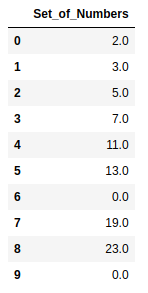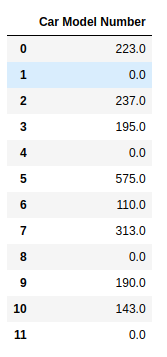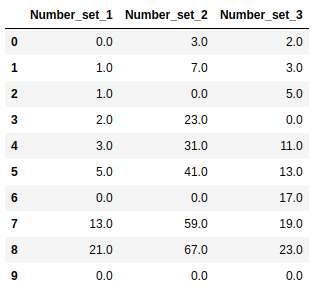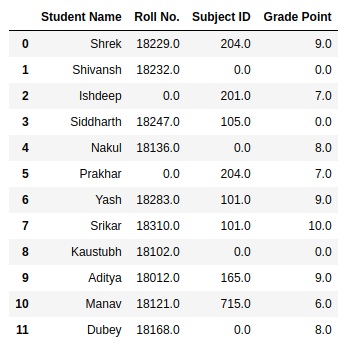# Replace NaN Values with Zeros in Pandas DataFrame

• Last Updated : 03 Jul, 2020

NaN stands for Not A Number and is one of the common ways to represent the missing value in the data. It is a special floating-point value and cannot be converted to any other type than float. NaN value is one of the major problems in Data Analysis. It is very essential to deal with NaN in order to get the desired results.Methods to replace NaN values with zeros in Pandas DataFrame:

• fillna()
The `fillna()` function is used to fill NA/NaN values using the specified method.

• replace()
The `dataframe.replace()` function in Pandas can be defined as a simple method used to replace a string, regex, list, dictionary etc. in a DataFrame.

Steps to replace NaN values:

• For one column using pandas:
```df['DataFrame Column'] = df['DataFrame Column'].fillna(0)
```
• For one column using numpy:
```df['DataFrame Column'] = df['DataFrame Column'].replace(np.nan, 0)
```
• For the whole DataFrame using pandas:
```df.fillna(0)
```
• For the whole DataFrame using numpy:
```df.replace(np.nan, 0)
```

Method 1: Using fillna() function for a single column

Example:

 `# importing libraries``import` `pandas as pd``import` `numpy as np`` ` `nums ``=` `{``'Set_of_Numbers'``: [``2``, ``3``, ``5``, ``7``, ``11``, ``13``, ``                           ``np.nan, ``19``, ``23``, np.nan]}`` ` `# Create the dataframe``df ``=` `pd.DataFrame(nums, columns ``=``[``'Set_of_Numbers'``])`` ` `# Apply the function``df[``'Set_of_Numbers'``] ``=` `df[``'Set_of_Numbers'``].fillna(``0``)`` ` `# print the DataFrame``df`

Output:#### Method 2: Using replace() function for a single column

Example:

 `# importing libraries``import` `pandas as pd``import` `numpy as np`` ` `nums ``=` `{``'Car Model Number'``: [``223``, np.nan, ``237``, ``195``, np.nan,``                             ``575``, ``110``, ``313``, np.nan, ``190``, ``143``, ``                             ``np.nan],``       ``'Engine Number'``: [``4511``, np.nan, ``7570``, ``1565``, ``1450``, ``3786``, ``                         ``2995``, ``5345``, ``7777``, ``2323``, ``2785``, ``1120``]}`` ` `# Create the dataframe``df ``=` `pd.DataFrame(nums, columns ``=``[``'Car Model Number'``])`` ` `# Apply the function``df[``'Car Model Number'``] ``=` `df[``'Car Model Number'``].replace(np.nan, ``0``)`` ` `# print the DataFrame``df`

Output:#### Method 3: Using fillna() function for the whole dataframe

Example:

 `# importing libraries``import` `pandas as pd``import` `numpy as np`` ` `nums ``=` `{``'Number_set_1'``: [``0``, ``1``, ``1``, ``2``, ``3``, ``5``, np.nan,``                         ``13``, ``21``, np.nan],``       ``'Number_set_2'``: [``3``, ``7``, np.nan, ``23``, ``31``, ``41``, ``                        ``np.nan, ``59``, ``67``, np.nan],``       ``'Number_set_3'``: [``2``, ``3``, ``5``, np.nan, ``11``, ``13``, ``17``,``                        ``19``, ``23``, np.nan]}`` ` `# Create the dataframe``df ``=` `pd.DataFrame(nums)`` ` `# Apply the function``df ``=` `df.fillna(``0``)`` ` `# print the DataFrame``df`

Output:#### Method 4: Using replace() function for the whole dataframe

Example:

 `# importing libraries``import` `pandas as pd``import` `numpy as np`` ` `nums ``=` `{``'Student Name'``: [ ``'Shrek'``, ``'Shivansh'``, ``'Ishdeep'``,  ``                         ``'Siddharth'``, ``'Nakul'``, ``'Prakhar'``,``                         ``'Yash'``, ``'Srikar'``, ``'Kaustubh'``, ``                         ``'Aditya'``,  ``'Manav'``, ``'Dubey'``],``        ``'Roll No.'``: [ ``18229``, ``18232``, np.nan, ``18247``, ``18136``, ``                     ``np.nan, ``18283``, ``18310``, ``18102``, ``18012``,``                     ``18121``, ``18168``],``        ``'Subject ID'``: [``204``, np.nan, ``201``, ``105``, np.nan, ``204``,``                       ``101``, ``101``, np.nan, ``165``, ``715``, np.nan],``       ``'Grade Point'``: [``9``, np.nan, ``7``, np.nan, ``8``, ``7``, ``9``, ``10``,``                       ``np.nan, ``9``, ``6``, ``8``]}`` ` `# Create the dataframe``df ``=` `pd.DataFrame(nums)`` ` `# Apply the function``df ``=` `df.replace(np.nan, ``0``)`` ` `# print the DataFrame``df`

Output:My Personal Notes arrow_drop_up